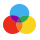Colors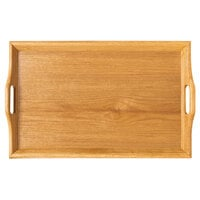From $26.68/Each Reg.Lots of 6$27.49$26.68$21.20/Each

From $16.68/Each Reg.Lots of 6$17.49$16.68 From$26.68/Each

Reg.Lots of 6
$27.49$26.68Colors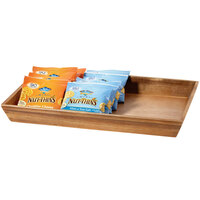From $25.17/Each 1 - 23+$25.99$25.17Colors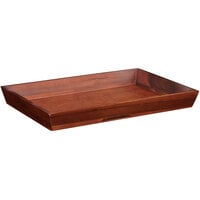From$25.17/Each

1 - 23+
$25.99$25.17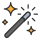Customizable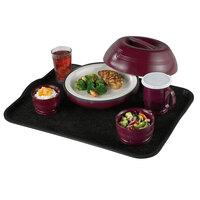From $272.46/Case 1 - 23+$282.49$272.46$53.49/EachColors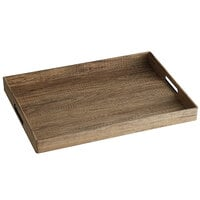$14.99/EachColors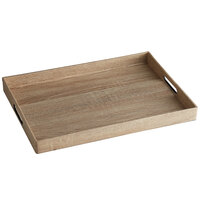$14.99/Each

$56.37/EachColors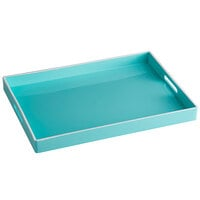$14.99/EachColors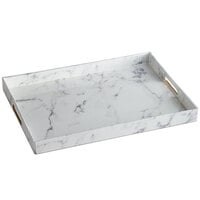$14.99/EachColors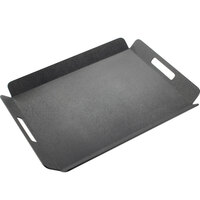$36.99/EachColors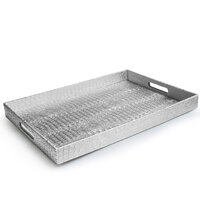$14.99/EachColors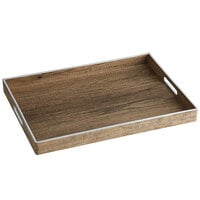$14.99/Each

From $11.49/Each Reg.Lots of 6$12.49$11.49$17.49/Each

$19.49/Each$17.49/EachColors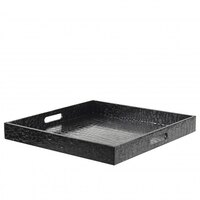$20.49/EachColors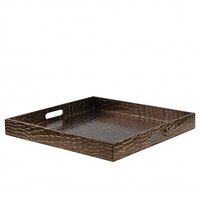$20.49/EachColors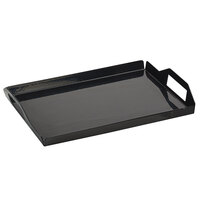$57.99/EachColors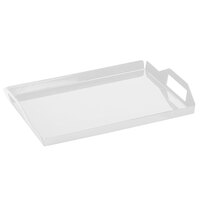$57.99/EachColors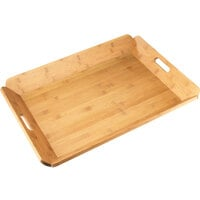$42.99/EachCustomizable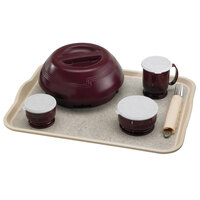From$272.46/Case

1 - 23+
$282.49$272.46

$53.79/Each From$32.43/Each

23+
$33.99$32.43